# Normal Neuron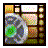## Watch the video tutorial on this subject!

### Neuron Properties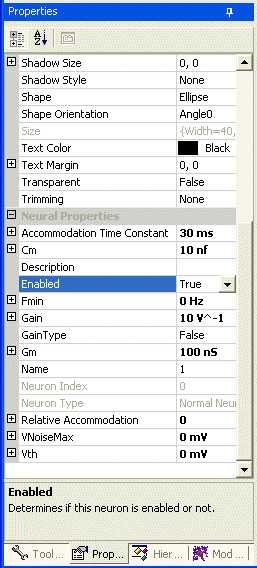Figure 1. Normal Neuron Properties

The normal firing rate neuron is the most basic type of neuron in the simulator system. All other neurons are derived from this one and have the basic functionality that this one displays. Figure 1 shows the properties toolbar and all of the basic variables that are associated with a normal neuron.

All the neurons in this module are derived from the Node object and share the properties of that item. For a description of these properties please see the text that discusses the Node properties.

Accommodation time constant
This sets the rate at which the threshold adjusts to its new value as the membrane potential changes.
Default value: 10 ms.
Acceptable range: 1 to 1000 ms.

Cm
The capacitance for the RC portion of this neuron.
Default value: 10 nf.
Acceptable range:Greater than 0.

Description
This is a text area where you can enter a detailed description of this neuron. When a user moves the mouse over this neuron a pop-up window will be briefly displayed that shows this descriptive text. This area is very useful for annotizing the parts of your circuit. For example, we are using it to keep track of which neurons in our circuits are based on real physiological data, and which are simply speculative. You can also add standard link descriptors in the text like ftp:\\, http:\\, and file:\\ for locations on your harddrive. This allows you to link a neuron or synapse to documents on the internet that describe it in detail.

Enabled
If this is false then it as if this neuron is no longer a part of the network. It does not process any incoming inputs and does not produce spikes or outputs.

Fmin
The minimum firing frequency. When the Vth value is reached by the membrane potential this is the frequency at which the neuron begins firing.
Default value: 0 Hz.
Acceptable range:Between 0 and 1.

Gain
Sets the gain of firing frequency for this neuron. The value used here depends on the gain type. I originally deviated from the description of the gain outlined in (Beer, 1991). So I had to support both methods to maintain backwards compatibility. Future projects should leave the gain type to be False to use the method outlined by Beer. With that version the gain is calculated using the following formula:

Freq = Fmin - Gain * Vth
if (Vm < (1 - Freq)/Gain)
Freq = (Gain * Vm) + Freq
else
Freq = 1

Default value: 0 mv-1.
Acceptable range:Greater than 0.

Gain Type
This should remain false for all new projects

Gm
The conductance for the RC portion of this neuron.
Default value: 100 nS.
Acceptable range:Greater than 0.

Name
This is the name of this neuron that will be displayed within its node in the diagram.

Neuron Index
This is a read-only index value used internally in the plug-in module.

Neuron Type
This is a read-only description of the type of this neuron. It can be spiking or non-spiking.

Relative accommodation
This sets the degree to which the spike threshold accommodates (increases) in response to depolarization. A value of 0 means no accommodation (threshold constant). A value of 1 means that when the membrane potential changes, the steady-state threshold (i.e. the threshold when the membrane potential has remained at its new level for a long period of time) exactly parallels the membrane potential: it remains as far above the membrane potential as it was in the resting condition.
Default value: 0.3.
Acceptable range: 0 to 1.

VNoiseMax
This causes random (uniform distribution) changes in membrane potential within the limits set by this parameter. This is useful to prevent metastable output patterns, and does not slow the simulation as much as using random synaptic input (see below).
Default value: 0 mV.
Acceptable range: 0 to 5 mV.

Vth
This is the threshold voltage. Voltage potentials below this value will not cause the neuron to fire. This model does not directly use the resting potential of the neuron. It treats changes in the membrane voltage as if they were changes from the resting potential. So you can think of a 10 mv positive change as being similar to a 10 mv depolarization from the resting potential of -70 mv.
Default value: 0 mV.
Acceptable range: Greater than 0.

### Neuron Output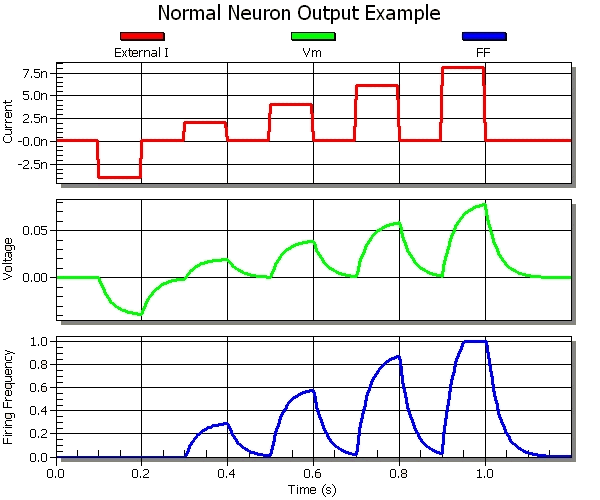Figure 2. This is a graph of the output from a normal neuron with externally applied input currents of -4 na, 2 na, 4 na, 6 na, and 8 na in 100 ms intervals with the following neuron properties: Cm = 3 nf, Gm = 100 nS, Vth = 0 mv, Fmin = 0 Hz, Gain = 15 V^-1

Figure 2 gives a demonstration of what the output looks like for a normal neuron when it is injected with different levels of current. When the graph initially begins there is no net input current into the neuron and the membrane voltage (Vm) is at 0. At 100 ms an external current of -4 na is applied and the membrane voltage dips to around -8 mV. This does not cause the neuron to fire because it will only fire if Vm >= Vth. Then at intervals an increasing amount of current is injected into the neuron and the voltage rises and falls, and the neuron fires once Vm passes the threshold. Also notice on the last current injection that the firing frequency saturated. It attempted to go above 1, but this is not allowed and the firing frequency peaked at this value and stayed there until membrane voltage fell.

### Modifying Cm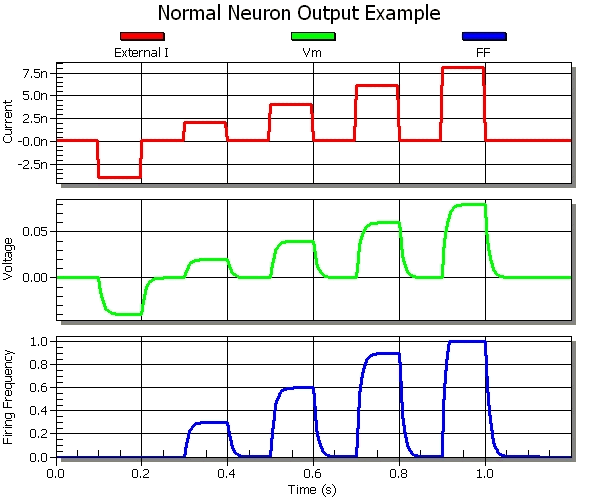Figure 3. This is a graph of the output from a normal neuron with externally applied input currents of 2 na, 4 na, 6 na, and 8 na in 100 msec intervals with the following neuron properties: Cm = 1 nf, Gm = 200 nS, Vth = 0 mv, Fmin = 0 Hz, Gain = 15 V^-1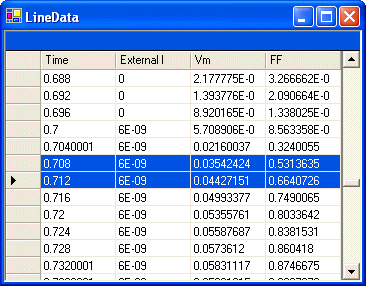Figure 4. This is the data for the chart in figure 3.

In Figure 3 the capacitance of the neuron was decreased from 3 nf to 1 nf. A very important property of RC circuits is the time constant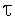= Rm * Cm = Cm / Gm. This value tells the time it will take for the voltage to increase by 63% when charging. This is demonstrated in figure 4. So as the capacitance is decreased the time it takes to charge that capacitor decreases. For instance, in the first example the circuit had Gm = 100 nS and Cm = 3 nf. This gives it a= 30 ms. For the new graph where we reduced Cm this value is= 1 nf/100 nS = 10 ms. If you are repeating these experiments in AnimatLab then right click on the chart to display the pop-up menu and select the "View Data" item. This will display the dialog from figure 4 that lists all the data points in the chart. First, if we look at 800 ms you will see that the max voltage for the 6 na current is 60 mv. So according to ourvalue the voltage should be V = 0.63 * 0.06 = 0.0378 = 38 mv at 10 ms. Our data does not actually stop at 10 ms, but by looking at the highlighted values in the data we can see that at 8 ms it is 35 mv and at 12 ms it is 44 ms. So it does go through 37 mv in the correct period.

Also, you can seen that even after 100 ms figure 2 is just reaching its steady state voltage level, but since we reduced the capacitance in figure 3 it was able to charge much faster and reached its steady state quickly. This is the major effect that can be seen from changing the capacitance of the neuron. Increasing the capacitance increases the time needed to charge / discharge the capacitor. This changes the responsiveness of a neuron.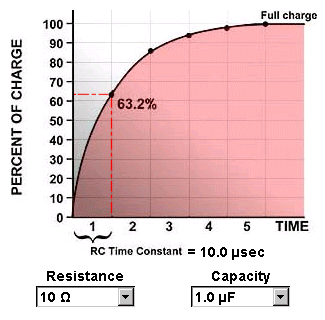Figure 5. This graph illustrates the time constant of an RC circuit. The first time constant location is shown with the dotted red line. Later time constants are shown with dots on the curve.

### Modifying Gm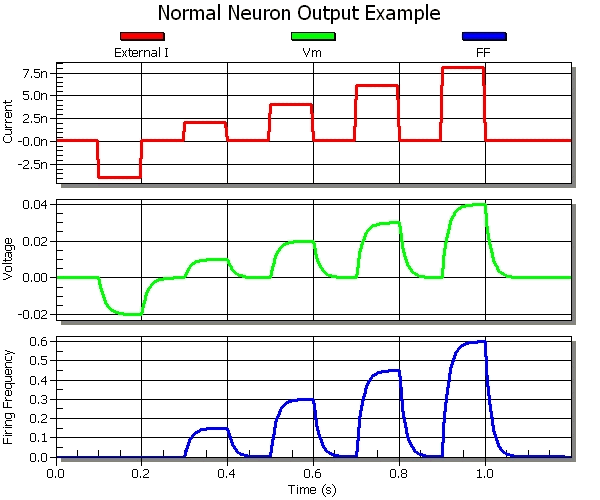Figure 6. This is a graph of the output from a normal neuron with externally applied input currents of -4 na, 2 na, 4 na, 6 na, and 8 na in 100 ms intervals with the following neuron properties: Cm = 3 nf, Gm = 200 nS, Vth = 0 mv, Fmin = 0 Hz, Gain = 15 V^-1

In figure 6 the conductance was increased from 100 nS to 200 nS relative to figure 2. Changing the conductance of a neuron has two major effects. First, it changes the time constant. The time constant for figure 2 is 30 ms, while= 15 ms for figure 6. This means that increasing the conductance decreases the amount of time it takes for the capacitor of the RC circuit to charge. The second major effect is related to the final steady state voltage. The steady state voltage is the voltage that results once the capacitor has been fully charged. The final membrane voltage is directly related to the input current and the conductance of the equation V = I * R. The capacitance has no relation to this final value. It only effects how long it takes to actually reach the value. In figure 2, an input current of 4 na causes a steady state voltage of 40 mV. In figure 6, an input current of 4 na causes a steady state voltage of 20 mV. This makes sense because since we doubled the conductance we halved the steady state voltage. This can easily be seen by comparing the amplitudes of both the membrane voltage and the firing frequencies from figures 2 and 6.

### Modifying Vth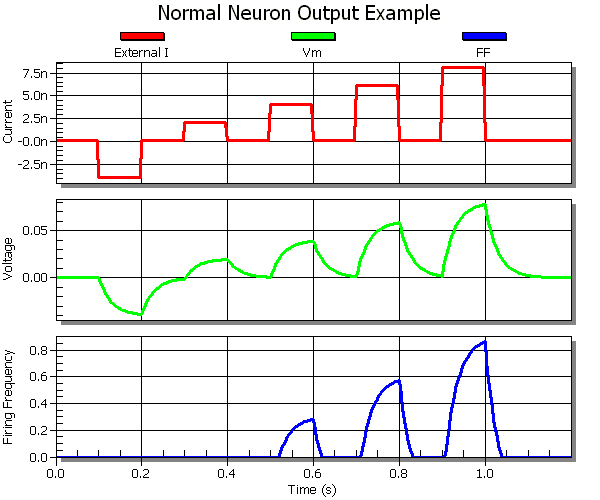Figure 7. This is a graph of the output from a normal neuron with externally applied input currents of -4 na, 2 na, 4 na, 6 na, and 8 na in 100 ms intervals with the following neuron properties: Cm = 3 nf, Gm = 100 nS, Vth = 20 mv, Fmin = 0 Hz, Gain = 15 V^-1

In figure 7 the threshold voltage was increased from 0 mV to 20 mV relative to figure 2. Right off the bat it can be seen that this caused the final firing frequency to be lower for all input currents. It also meant that the neuron did not even start firing until after it was injected with 4 na or higher. The reason for this is because the 2 na current injection was no longer sufficient to produce a membrane voltage over the 20 mV level. This means it did not exceed the threshold and thus the neuron did not fire. The reason that the final amplitude of the firing frequency for the other current injections were lower is because the firing frequency is based on the difference from the threshold, not the difference from the 0 mV level. So even though an injection of 4 na caused a membrane voltage of 40 mV, this was only 20 mV above the threshold and so it was treated as if it was 20 mV.

### Modifying Fmin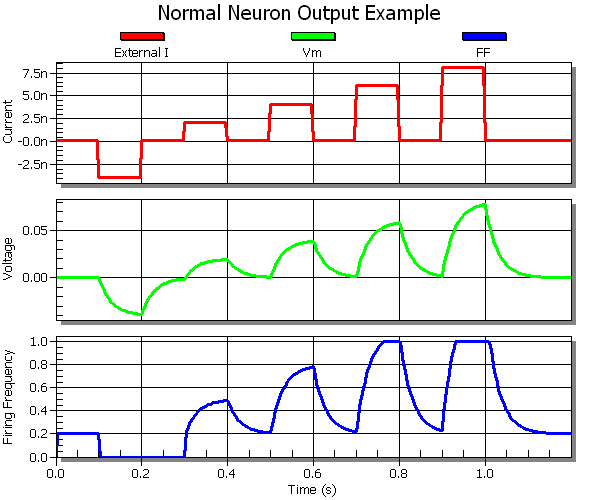Figure 8. This is a graph of the output from a normal neuron with externally applied input currents of -4 na, 2 na, 4 na, 6 na, and 8 na in 100 ms intervals with the following neuron properties: Cm = 3 nf, Gm = 100 nS, Vth = 0 mv, Fmin = 0.2 Hz, Gain = 15 V^-1

In figure 8 the minimum firing frequency was increased from 0 Hz to 0.2 Hz relative to figure 2. The first noticeable change is that the neuron is firing at 0.2 Hz even though no current has been injected and the membrane voltage is at 0 mV. This is because the firing threshold is set at 0 mV. Once the negative current is injected and the membrane potential falls below zero it can be seen that the firing frequency is clamped back down to zero. It does not return back to the minimum firing until the 2 na current is injected because the voltage is still discharging back to its 0 v level. Once the positive currents are injected it is as if the output from figure 2 was taken and simply shifted up by 0.2 Hz. This is in affect what has been done. When the capacitor discharges it returns not to a zero firing frequency as before, but instead falls to a 0.2 Hz firing frequency. This is the major effect caused by changing the minimum firing frequency. By playing with this value and the threshold simultaneously it is possible to build a neuron that spontaneously, and continuously fires at a given rate unless it is actively inhibited.

### Modifying Gain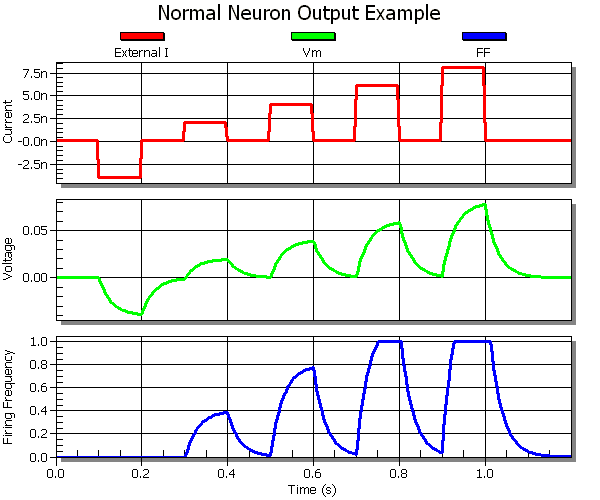Figure 9. This is a graph of the output from a normal neuron with externally applied input currents of -4 na, 2 na, 4 na, 6 na, and 8 na in 100 ms intervals with the following neuron properties: Cm = 3 nf, Gm = 100 nS, Vth = 0 mv, Fmin = 0 Hz, Gain = 20 V^-1

In figure 9 the gain was increased from 15 V^-1 to 20 V^-1 relative to figure 2. The gain is basically just the slope of the line of the function that relates membrane voltage to firing frequency. Increasing the gain means increasing the firing frequency of the neuron for the same membrane potential. And decreasing the gain does the exact opposite. This can be seen by comparing figures 9 and 2. The steady state firing frequency for the 4 na input current is around 0.6 Hz for figure 2, but it is around 0.80 Hz for figure 9. Otherwise the graphs are the same. So gain makes the firing frequency of the neuron more or less sensitive to the membrane voltage.

### Neuron Property Overview

The preceding sections have demonstrated some of the effects that can be obtained by modifying each of the different properties of the model neuron. This was done by using a base neuron with standard parameters and then modifying one of the values to see what effect this had on the output. Once these basic effects are understood it is then possible to begin putting together multiple changes to try and produce neurons that will behave in the desired manner. Without this understanding it will be very difficult for the experimenter to understand what parameters need to be tuned in order to get a specific behavior of the organism to work correctly. So a good insight into what each of these properties do is critical to really beginning to understand what is happening in the overall network of neurons.